# Robotics Toolbox#

## 机器人模型建立#

``````L1 = Link('d', 0, 'a', 0, 'alpha', pi/2);
L2 = Link('d', 0, 'a', 0.5, 'alpha', 0,'offset',pi/2);
L3 = Link('d', 0, 'a', 0, 'alpha', pi/2,'offset',pi/4);
L4 = Link('d', 1, 'a', 0, 'alpha', -pi/2);
robot.name='testRobot';
robot.display();
theta=[0 0 0 0];
robot.plot(theta); %显示机器人的图像
``````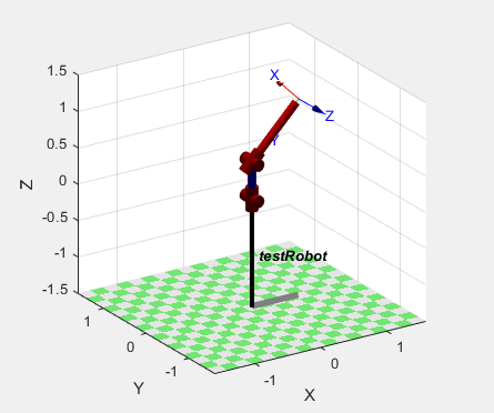机器人模型建立

``````L1 = Link('d', 0, 'a', 0, 'alpha', pi/2);
L2 = Link('d', 0, 'a', 0, 'alpha', pi/2);
L3 = Link('d', 0, 'a', 0, 'alpha', pi/2);
L4 = Link('d', 0.5, 'a', 0, 'alpha', 0);
robot.name='testRobot';
robot.display();
theta=[0 0 0 0];
robot.teach(theta); %显示机器人的图像
``````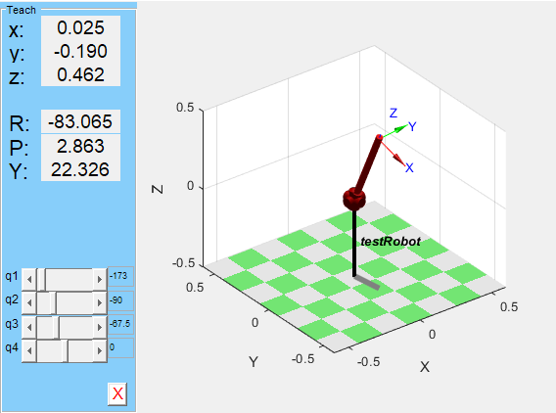球坐标机器人示教

PUMA560 作为一个经典的模型，已经被工具箱作为标准机器人收录，可以使用如下命令直接调出：`mdl_puma560`，同样使用`teach`进行示教效果如下。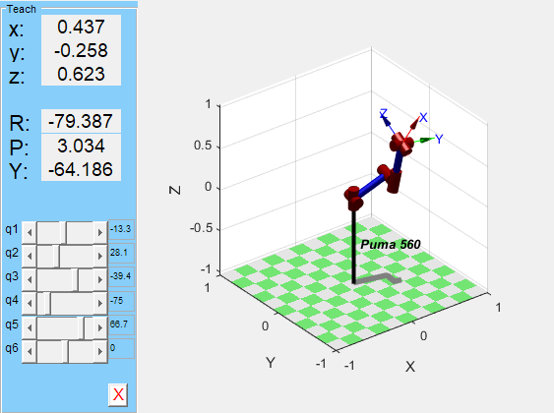PUMA560 示教

## 正逆运动学求解#

``````target=[1,1,1,1,1,1];
%target=[0,pi/3,-pi/3,0,pi/4,0];
fkine(p560,target);
p560.plot(target);
``````

``````target=[pi/4,-pi/3,0,0,0,pi/6];
p1=p560.fkine(target);
q1=p560.ikine(p1);
``````

# 基本轨迹规划#535 多项式轨迹规划

# 水刀运动学模型#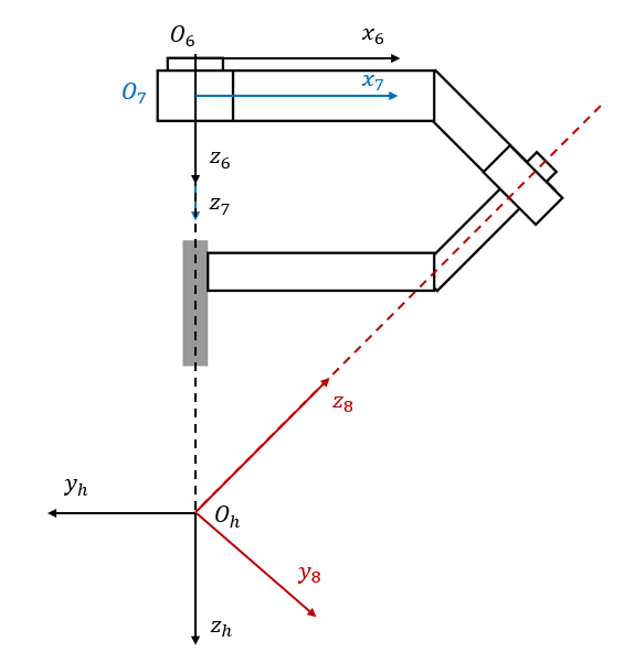水刀建模

Joint numaidiαiθi
6-700.0100
7-800.133/4ππ/2+β
8-h00-3/4πθ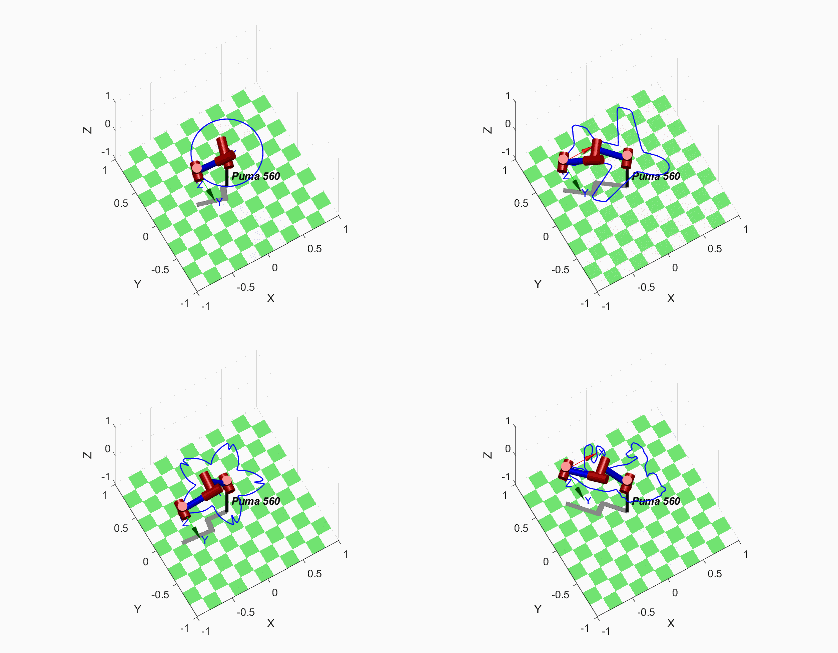水刀机架轨迹规划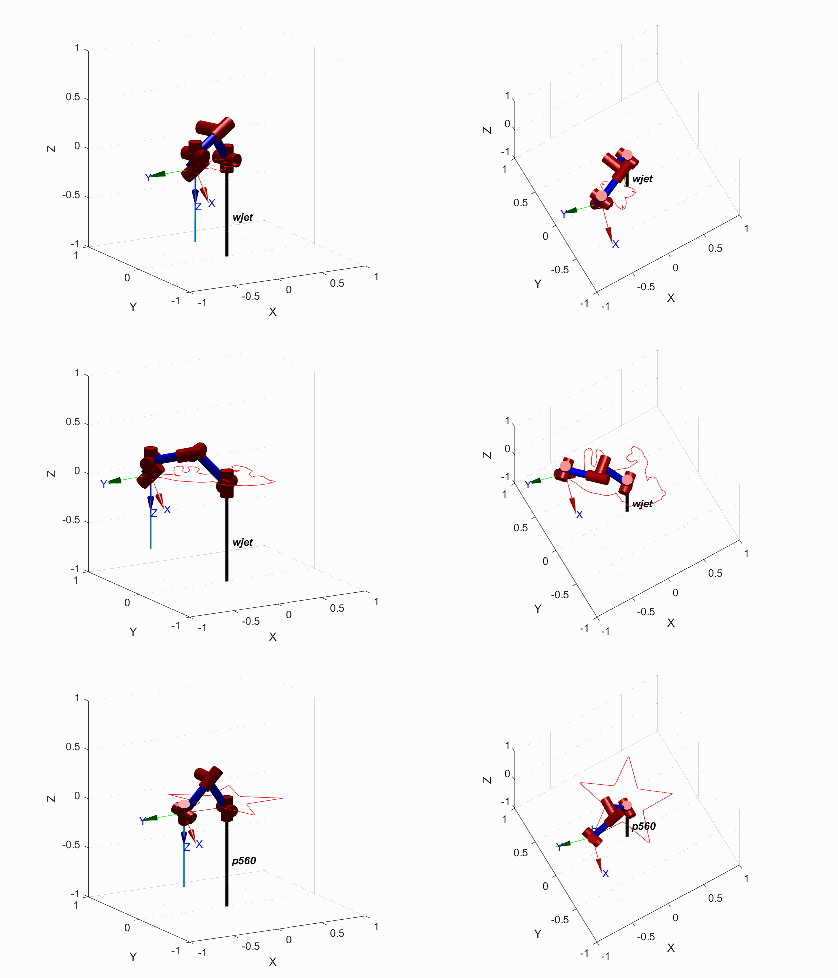水刀切割动画

# 参考资料#

1. 《机器人学导论：分析、控制及应用》Saeed B.Niku
2. Matlab 中 Robotics toolbox 的安装及使用
3. ikine6s.m robotics-toolbox-matlab GitHub